# Mass

The thickness of a metallic tube is 1cm, and its outer radius is 11cm. Find the mass of such a 1m long tube if the metal density is 7.5g per cubic cm.

m =  49.48 kg

### Step-by-step explanation: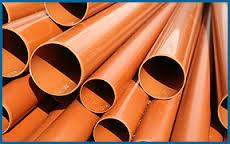Did you find an error or inaccuracy? Feel free to write us. Thank you!Tips to related online calculators
Tip: Our Density units converter will help you with the conversion of density units.
Do you want to convert mass units?

## Related math problems and questions:

• Metal tubeCalculate the metal tube mass 8dm long with the outer radius 5cm and the inner radius 4.5cm and 1cm3 of this metal is 9.5g.
• Brass tubeThe outer perimeter of brass tube (ρ = 8.5 g/cm3) is 38 cm. Its mass is 5 kg, length 54 cm. What is the pipe wall thickness?
• Steel tubeThe steel tube has an inner diameter of 4 cm and an outer diameter of 4.8 cm. The density of the steel is 7800 kg/m3. Calculate its length if it weighs 15 kg.
• Iron densityCalculate the weight of a 2 m long rail pipe with an internal diameter of 10 cm and a wall thickness of 3 mm. The iron density is p = 7.8 g/cm3.
• Wall thicknessThe hollow metal ball has an outside diameter of 40 cm. Determine the wall thickness if the weight is 25 kg and the metal density is 8.45 g/cm3.
• Brass sphereFind the weight of a brass ball with an outer radius of 12 cm, a wall thickness of 20 mm if the brass's density is 8.5 g/cm3.
• The pipe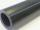The pipe is 1.5 m long. Its outer diameter is 60 cm, inner diameter is 52 cm. Calculate the pipe's weight if the material's density from which it is made is 2 g/cm3. Round the results to whole kilograms.
• LidWhat is the weight of concrete cover (lid) to round shape well with a diameter 1.8 m, if the thickness of the cover is 11 cm? 1 m3 of concrete weighs 2190 kg.
• Brass ball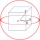What is the weight of a hollow brass ball, the outer diameter of which is 16 cm and the wall thickness is 2 cm, while the density of brass is ϱ = 8.5 g
• Metal sheets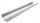How much metal sheet is needed to produce 8 gutters 4 m long and 12 cm in diameter? During production, joints calculate at 3% of total consumption.
• The glass1 m3 of glass weighs 2600 kg. Calculate the weight of the glass glazing panel with dimensions of 2.5 m and 3.8 m if the thickness of the glass is 0.8 cm
• SnowSnow fell overnight layer of thickness 19 cm. In the morning I had to clear a path 69 m long and one meter wide. How many cubic meters of snow I clear? How many kilos was it? (1 m3 fresh snow weighs 350 kg)
• Giant coin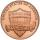From coinage metal was produced giant coin and was applied so much metal, such as production of 10 million actual coins. What has this giant coin diameter and thickness, if the ratio of diameter to thickness is the same as a real coin, which has a diamete
• Plastic pipeCalculate the plastic pipe's weight with diameter d = 70 mm and length 380 cm if the wall thickness is 4 mm and the density of plastic is 1367 kg/m3.
• Brick wallWhat is the weight of a solid brick wall that is 30 cm wide, 4 m long and 2 m high? The density of the brick is 1500 kg per cubic meter.
• Copper wire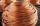What is the weight of 1000 m copper wire with a diameter of 5 mm when metric density p = 8.8 g/cm3?
• Copper plateCalculate the thickness of the copper plate with a density 8.7 g/cm³ measuring 1.5 meters and 80 cm and its weight is 3.65 kg